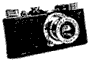Archived posting to the Leica Users Group, 2004/07/07

[Author Prev] [Author Next] [Thread Prev] [Thread Next] [Author Index] [Topic Index] [Home] [Search]

Subject: [Leica] "focus then reframe" (aka fungus in camera)
From: jean.louchet at inria.fr (Jean Louchet)
Date: Wed Jul 7 14:25:50 2004

```Hi all,

Beware, the calculation maniac is back on line :-)

Apart the Descartes formula, the second important formula is the "Setala
formula" which allows to calculate the depth of field, the diameter of the
blur circle etc. (According to the "photo club de Bievres", Mr Setala was
a Leica User from Finland, and the inventor of the "depth of focus" scales
one now finds on almost all lenses)

Its parameters are:
the diameter of the blur circle: Delta
the diameter of the diaphragm:   Diaph
the focal distance: f
the distance on which the lens is focussed: z
the actual object's distance: d

Setala's formula is:
Delta = Diaph * f * abs(1/z - 1/d)

It is only true when z is much greater than f (always the case except in
macrophotography)

Let's take as an example a 35mm lens on a leica. As the focal length f
(35mm) is about equal to the width of the image (36mm), if we want to
photograph a vertical wall at distance d, the part of the wall which
projects onto the side of the picture will be at an actual distance z
(measured by the rangefinder before turning the camera) such that

d^2 = z^2 + e^2 where e is half the width of the part of the wall that
will be visible on the picture (sorry I can't make a drawing here, but
this is Pythagore's theorem), and e=z/2 (because we have a 35mm lens and a
18mm half-width of the picture).  Thus we can calculate 1/z - 1/d,
eliminating d (d = z * (sqrt(5)/2 = z*1.118) gives
1/z - 1/d = 1/z *(1 - 2/sqrt(5)) = 0.105/z

At aperture f/2 we have (in millimeters)
Diaph = 17.5mm
and
Delta = 17.5 * 35 * 0.105 * (1/z)  (z also in millimeters!)
Delta = 64.3/z

This is the diameter of the "blurring" on the picture of the wall if we
rely on the "focus then reframe" technique.

At infinity (z = inf) the picture will be (in theory) perfectly sharp.
At z = 10 metres we have Delta = 64.3/10000 = 6.4 microns = perfectly
sharp in practice.
At z = 5 metres, Delta = 13 microns = very sharp (would still resolve 80
lines/mm).
At z = 2 metres, Delta = 32 microns = usually considered "good" or
"acceptable" (30 lines/mm).
At z - 1 metre, Delta = 64 microns :-( it is worth to make the focussing
correction.

The correction coefficient on the edges is 1.118 (say 12%). If one uses
the rangefinder on an object and wants this object to be sharp when it
gets on the side of the picture, he will have to SUBTRACT 12% to the
distance given by the rangefinder.

The same formulas with aperture 1.4 would give:
At z = 10 metres Delta = 9 microns "very sharp"
At z = 5 metres Delta = 18 microns "sharp"
At z = 2 metres Delta = 45 microns "fair" :-|

TO SUMMARISE:
with a 35mm, at aperture 2 "focus then reframe" is OK from 2 metres
at aperture 1.4 "FTR" is OK from 3 metres.

At shorter distances, one has to reduce the focussing by, say, 10 - 12%.

I did not consider focussing on an object which will finally be right in a
corner (no practical interest).

OF COURSE we luggers will take much, much better pictures now :-)

If anyone is interested in having the equivalent results with a 50mm, let
me know (private e-mail), I may well open another page on my web site.

Jean

--
------------------------------------------------------------
Dr Jean Louchet       COMPLEX Project     INRIA Rocquencourt
BP105   78153 Le Chesnay cedex, France
Jean.Louchet@inria.fr     http://fractales.inria.fr/~louchet
+33 (0)1 3963 5582/5104              fax: +33 (0)1 3963 5995
------------------------------------------------------------

```

Replies: Reply from henningw at archiphoto.com (Henning Wulff) ([Leica] "focus then reframe" (aka fungus in camera))
Reply from richard-lists at imagecraft.com (Richard F. Man) ([Leica] "focus then reframe" (aka fungus in camera))
Reply from images at InfoAve.Net (Tina Manley) ([Leica] "focus then reframe" (aka fungus in camera))